Courses

# Instrumentation Engineering - IN 2015 GATE Paper (Practice Test)

## 65 Questions MCQ Test GATE Past Year Papers for Practice (All Branches) | Instrumentation Engineering - IN 2015 GATE Paper (Practice Test)

Description
This mock test of Instrumentation Engineering - IN 2015 GATE Paper (Practice Test) for GATE helps you for every GATE entrance exam. This contains 65 Multiple Choice Questions for GATE Instrumentation Engineering - IN 2015 GATE Paper (Practice Test) (mcq) to study with solutions a complete question bank. The solved questions answers in this Instrumentation Engineering - IN 2015 GATE Paper (Practice Test) quiz give you a good mix of easy questions and tough questions. GATE students definitely take this Instrumentation Engineering - IN 2015 GATE Paper (Practice Test) exercise for a better result in the exam. You can find other Instrumentation Engineering - IN 2015 GATE Paper (Practice Test) extra questions, long questions & short questions for GATE on EduRev as well by searching above.
QUESTION: 1

Solution:
QUESTION: 2

Solution:
QUESTION: 3

### Choose the appropriate word/phrase, out of the four options given below, to complete the following sentence: Apparent lifelessness _______________ dormant life.

Solution:
QUESTION: 4

Fill in the blank with the correct idiom/phrase.

That boy from the town was a __________in the sleepy village.

Solution:
QUESTION: 5

Tanya is older than Eric.

Cliff is older than Tanya.

Eric is older than Cliff.

If the first two statements are true, then the third statement is:

Solution:
QUESTION: 6

Q. No. 6 – 10 Carry Two Marks Each

Given below are two statements followed by two conclusions. Assuming these statements to be true decide which one logically follows.

Statement:

1. No manager is a leader.

Conclusions:

1. No manager is an executive.

2. No executive is a manager.

Solution:
QUESTION: 7

Select the appropriate option in place of underlined part of the sentence.

Increased productivity necessary reflects greater efforts made by the employees.

Solution:
*Answer can only contain numeric values
QUESTION: 8

In the given figure angle Q is a right angle, PS:QS = 3:1, RT:QT = 5:2 and PU:UR =1:1

If area of triangle QTS is 20 cm2, then the area of triangle PQR in cm2 is _______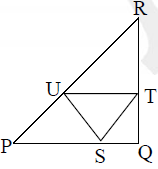Solution:
QUESTION: 9

Right triangle PQR is to be constructed in the xy-plane so that the right angle is at P and line PR is parallel to the x-axis. The x and y coordinate of P.Q, and R are to be integers that satisfy the inequalities:

–4 < x < 5 and 6 < y < 16. How many different triangles could be constructed with these properties?

Solution:
QUESTION: 10

A coin is tossed thrice. Let X be the event that head occurs in each of the first two tosses. Let Y be the event that a tail occurs on the third toss. Let Z be the event that two tails occur in three tosses. Based on the above information, which one of the following statements is TRUE?

Solution:
QUESTION: 11

Q. 11 – Q. 35 carry one mark each.

A load resistor RL is connected to a battery of voltage E with internal resistance Ri through a resistance RS a shown in the figure. For fixed values of RL and Ri, the value of RS (> 0) for maximum power transfer to RL is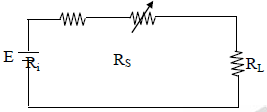Solution:
*Answer can only contain numeric values
QUESTION: 12

A system with transfer function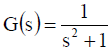has zero initial conditions. The percentage overshoot in its step response is ___________ %

(Important - Enter only the numerical value in the answer)

Solution:
*Answer can only contain numeric values
QUESTION: 13

A light detector circuit using an ideal photodiode is shown in the figure. The sensitivity of the photo-diode is 0.5μA/μW, with Vr = 6V, the output voltage Vo = – 1.0V for 10 μw of incident light. If Vr is changed to 3 V, keeping all other parameters the same, the value of Vo in volts is ___________ V.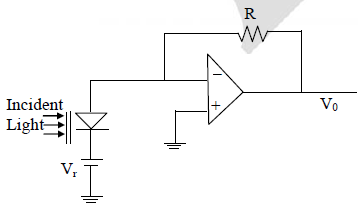(Important - Enter only the numerical value in the answer)

Solution:
*Answer can only contain numeric values
QUESTION: 14

The figure show a half-wave rectifier circuit with input voltage V(t) = 10 sin (100 π t) volts. Assuming ideal diode characteristics with zero forward voltage drop and zero reverse current, the average power consumed in watts by the load resistance RL is ________ w.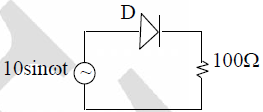(Important - Enter only the numerical value in the answer)

Solution:
QUESTION: 15

The bridge most suited for measurement of a four-terminal resistance in the range of 0.001? to 0.1 ? is

Solution:
QUESTION: 16

The voltage (Eo) developed across a glass electrode for pH measurement is related to the temperature (T) by the relation

Solution:
QUESTION: 17

The output voltage of the ideal transformer with the polarities and dots shown in the figure is given by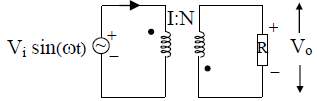Solution:
QUESTION: 18

Consider the logic circuit with input signal TEST shown in the figure. All gates in the figure shown have identical non-zero delay. The signal TEST which was at logic LOW is switched to logic HIGH. The output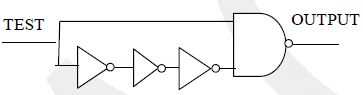Solution:
QUESTION: 19

The magnitude of the directional derivative of the function f(x,y)= x+ 3y2 in a direction normal to the circle x2 + y2 =2, at the point (1,1), is

Solution:
QUESTION: 20

The logic evaluated by the circuit at the output is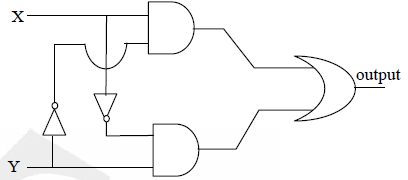Solution:
QUESTION: 21

The filter whose transfer function is of the form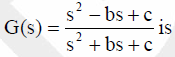Solution:
QUESTION: 22

Let A be an n x n matrix with rank r (0 < r < n). Then AX = 0 has p independent solutions, where p is

Solution:
QUESTION: 23

Liquid flow rate is measured using

Solution:
QUESTION: 24

The double integral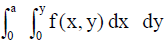is equivalent to

Solution:
*Answer can only contain numeric values
QUESTION: 25

The capacitor shown in the figure is initially charged to +10 V. The switch closes at time t = 0. Then the value of VC(t) in volts at time t = 10 ms is _____ V.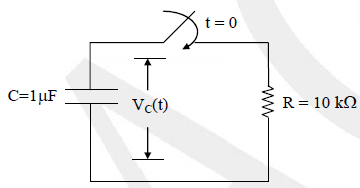(Important - Enter only the numerical value in the answer)

Solution:
QUESTION: 26

An apparatus to capture ECG signals has a filter followed by a data acquisition system. The filter best suited for this application is

Solution:
*Answer can only contain numeric values
QUESTION: 27

Consider the ammeter-voltmeter method of determining the value of the resistance R using the circuit shown in the figure. The maximum possible errors of the voltmeter and ammeter are known to be 1% and 2% of their readings, respectively. Neglecting the effects of meter resistances, the maximum possible percentage error in the value of R determined from the measurements, is _____ %.

(Important - Enter only the numerical value in the answer)

Solution:
QUESTION: 28

A mass-spring-damper system with force as input and displacement of the mass as output has a transfer function G(s) = 1/(s2+24s+900). A force input F(t) = 10 sin(70t) Newton is applied at time t = 0s. a beam from an option stroboscope is focused on the mass. In steady state, the strobe frequency in hertz at which the mass appears to be stationary is

Solution:
QUESTION: 29

The torque transmitted by a cylindrical shaft is to be measured by using two strain gauges. The angles for mounting the strain gauges relative to the axis of the shaft for maximum sensitivity are

(Important - Enter only the numerical value in the answer)

Solution:
*Answer can only contain numeric values
QUESTION: 30

A p–type semiconductor strain gauge has a nominal resistance of 1000 ? and a gauge factor of +200 at 25oC. The resistance of the strain gauge in ohms when subjected to a strain of +10–4 m/m at the same temperature is _______ ?.

(Important - Enter only the numerical value in the answer)

Solution:
QUESTION: 31

A power line is coupled capacitively through various parasitic capacitances to a shielded signal line as shown in the figure. The conductive shield is grounded solidly at one end. Assume that the length of the signal wire extending beyond the shield, and the shield resistance are negligible. The magnitude of the noise voltage coupled to the signal line is

Solution:
QUESTION: 32

In the circuit shown, the switch is momentarily closed and then opened. Assuming the logic gates to have equal nonzero delay, at steady state, the logic states of X and Y are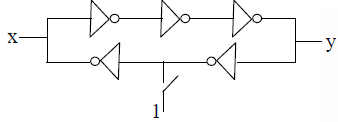Solution:
QUESTION: 33

Let 3 + 4j be zero of a fourth order linearphase FIR filter. The complex number which is NOT a zero of this filter is

Solution:
QUESTION: 34

The value of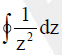, where the contour is the unit circle traversed clockwise, is

Solution:
QUESTION: 35

The highest frequency present in the signal x(t) is fmax. The highest frequency present in the signal y(t) = x2(t) is

Solution:
*Answer can only contain numeric values
QUESTION: 36

Q. No. 36 – 65 Carry Two Marks Each

In the circuit shown in the figure, it is found that VBE = 0.7 and VE = 0 V. If βdc = 99 for the transistor, then the value of RB in kilo ohms is _______ k?.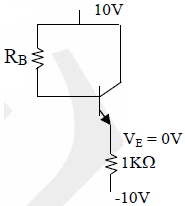(Important - Enter only the numerical value in the answer)

Solution:
QUESTION: 37

Consider a low-pass filter module with a pass-band ripple of δ in the gain magnitude. If M such identical modules are cascaded, ignoring the loading effects, the pass-band ripple of the cascade is

Solution:
*Answer can only contain numeric values
QUESTION: 38

A liquid level measurement system employing a radio-isotope is mounted on a tank as shown in the figure. The absorption coefficient of water for the radiation is 7.7 m–1. If the height of water in the tank is
reduced from 100 mm to 90 mm, the percentage change in the radiation intensity received by the detector, neglecting absorption of the radiation by air, is _____%.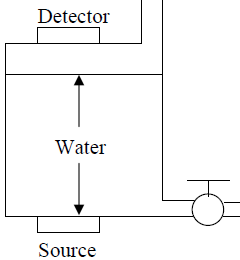(Important - Enter only the numerical value in the answer)

Solution:
*Answer can only contain numeric values
QUESTION: 39

The current in amperes through the resistor R in the circuit shown in the figure is _____ A.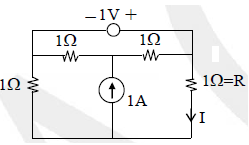(Important - Enter only the numerical value in the answer)

Solution:
*Answer can only contain numeric values
QUESTION: 40

The probability density function of a random variable X is px(x) = e–x for x > 0 and 0 otherwise. The expected value of the function gx(x) = e3x/4 is ________.

(Important - Enter only the numerical value in the answer)

Solution:
QUESTION: 41

The resolving power of a spectrometer consisting of a collimator, a grating a telescope can be increased by

Solution:
*Answer can only contain numeric values
QUESTION: 42

In the figure shown, RT represents a resistance temperature device (RTD), whose characteristic is given by RT = Ro(1+αT), where Ro = 100 ?, α = 0.0039oC–1 and T denotes the temperature in oC. Assuming the
op-amp to be ideal, the value of V0 in volts when T = 100oC, is _____ V.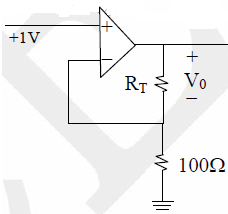(Important - Enter only the numerical value in the answer)

Solution:
*Answer can only contain numeric values
QUESTION: 43

The output frequency of an LC tank oscillator employing a capacitive sensor acting as the capacitor of the tank is 100 kHz. If the sensor capacitance increases by 10%, the output frequency in kilo-hertz becomes _______kHz.

(Important - Enter only the numerical value in the answer)

Solution:
*Answer can only contain numeric values
QUESTION: 44

A system is represented in state-space as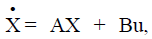where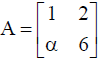and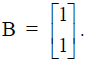The value of α for which the system is not controllable is _______.

(Important - Enter only the numerical value in the answer)

Solution:
*Answer can only contain numeric values
QUESTION: 45

The circuit shown in the figure is in series resonance at frequency fc Hz. The value of Vc in volts _______ V.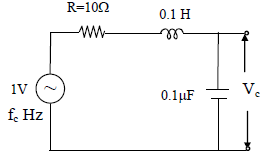(Important - Enter only the numerical value in the answer)

Solution:
*Answer can only contain numeric values
QUESTION: 46

The Seebeck coefficients, inμV/oC, for copper, constantan and iron, with respect to platinum, are 1.9, – 38.3 and 13.3, respectively. The magnitude of the thermo emf E developed in the circuit shown in the figure, in millivolts is ______ mV.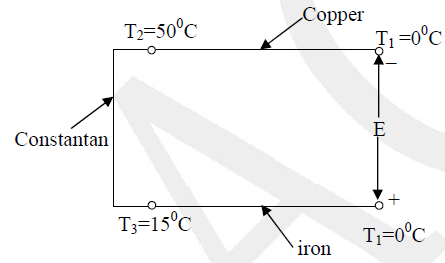(Important - Enter only the numerical value in the answer)

Solution:
QUESTION: 47

The z-transform of x[n] =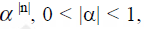is X(z). The region of convergence of X(z) is

Solution:
*Answer can only contain numeric values
QUESTION: 48

If the deflection of the galvanometer in the bridge circuit shown in the figure is zero, then the value of Rx in ohms is ______ ?.

(Important - Enter only the numerical value in the answer)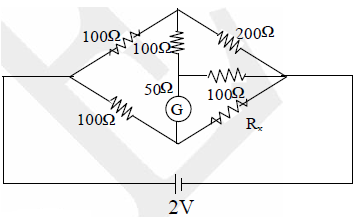Solution:
QUESTION: 49

An ADC is interfaced with a microprocessor as shown in the figure. All signals have been indicated with typical
notations. Acquisition of one new sample of the analog input signal by the microprocessor involves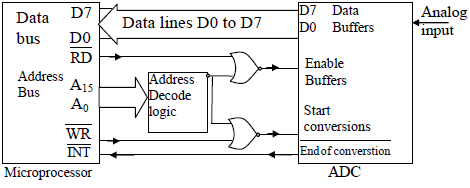Solution:
*Answer can only contain numeric values
QUESTION: 50

In the circuit shown in the figure, both the NMOS transistors are identical with their threshold voltages being 5 V. Ignoring channel length modulation, the output voltage Vout in volt is ______ V.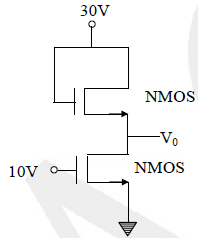(Important - Enter only the numerical value in the answer)

Solution:
QUESTION: 51

The probability that a thermistor randomly picked up from a production unit is defective is 0.1. The probability that out of 10 thermistors randomly picked up, 3 are defective is

Solution:
QUESTION: 52

A transfer function G(s) with the degree of its numerator polynomial zero and the degree of its denominator polynomial two has a Nyquist plot shown in the figure. The transfer function represents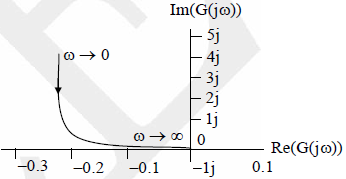Solution:
*Answer can only contain numeric values
QUESTION: 53

The linear I–V characteristics of 2–terminal non-ideal sc sources X and Y are shown in the figure. If the sources are connected to a 1? resistor as shown, the current through the resistor in amperes is ______ A.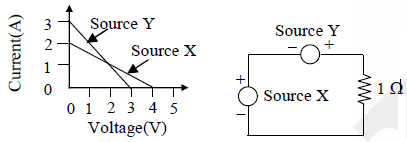(Important - Enter only the numerical value in the answer)

Solution:
*Answer can only contain numeric values
QUESTION: 54

Consider the circuits shown in the figure. The magnitude of the ratio of the currents, i.e., |I1/I2|, is ________.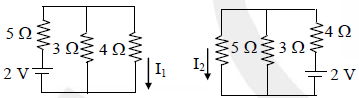(Important - Enter only the numerical value in the answer)

Solution:
QUESTION: 55

The open loop transfer function of a system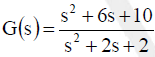. The angle of arrival of its root loci are

Solution:
QUESTION: 56

The signal x[n]= sin ( πn/6)/ (πn) is processed through a linear filter with the impulse response h[n] = sin(ωcn)/ (πn) where ωc > π/6. The output of the filter is

Solution:
QUESTION: 57

In the potentiometer circuit shown in the figure, the expression for Vx is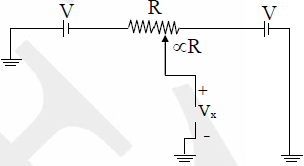Solution:
*Answer can only contain numeric values
QUESTION: 58

The figure shows a spot of light of uniform intensity 50 W/m2 and size 10 mm x 10 mm incident at the exact center of a photodetector, comprising two identical photodiodes D1 and D2 Each diode has a sensitivity of 0.4 A/W and is operated in the photoconductive mode. If the spot of light is displaced upwards by 100 μm, the resulting difference between the photocurrents generated by D1 and D2 in micro amperes, is _______ μA.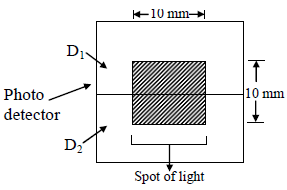(Important - Enter only the numerical value in the answer)

Solution:
QUESTION: 59

The number of clock cycles for the duration of an input pulse is counted using a cascade of N decade counters (DC 1 to DC N) as shown in the figure. If the clock frequency in mega hertz is f, the resolution and range of measurement of input pulse width, both in μs, are respectively,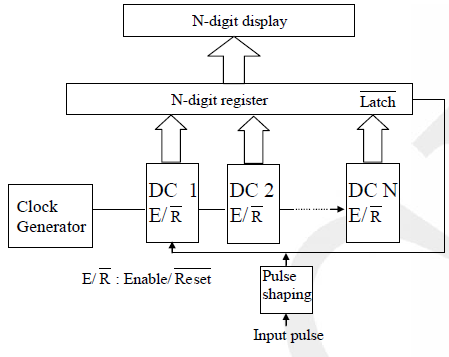(Important - Enter only the numerical value in the answer)

Solution:
*Answer can only contain numeric values
QUESTION: 60

A signal is band-limited to 0 to 12 kHz. The signal spectrum is corrupted by additive noise which is band-limited to 10 to 12 kHz. Theoretically, the minimum rate in kilohertz at which the noisy signal must be sampled
so that the UNCORRUPTED PART of the signal spectrum can be recovered, is ____ kHz.

(Important - Enter only the numerical value in the answer)

Solution:
*Answer can only contain numeric values
QUESTION: 61

A beam of monochromatic light passes through two glass slabs of the same geometrical thickness at normal incidence. The refractive index of the first slab is 1.5 and that of the second, 2.0. The ratio of the time of passage of the beam through the first to the second slab is __________ .

(Important - Enter only the numerical value in the answer)

Solution:
*Answer can only contain numeric values
QUESTION: 62

The fundamental period of the signal x(t) =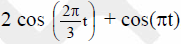, in second, is ____ s.

(Important - Enter only the numerical value in the answer)

Solution:
QUESTION: 63

For the circuit shown in the figure, the raising edge triggered D –flip flop with asynchronous reset has a clock frequency of 1 Hz . The NMOS transistor has an ON resistance of 1000 ? and an OFF resistance of infinity. The nature of the output waveform is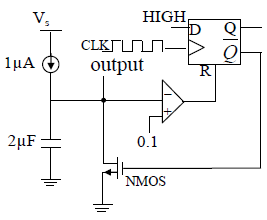Solution:
QUESTION: 64

An op-amp has ideal characteristics except that its open loop gain is given by the expression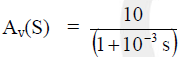. This opamp is used in the circuit shown in the figure. The 3-dB bandwidth of the circuit, in rad/s, is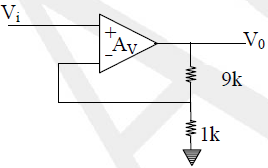(Important - Enter only the numerical value in the answer)

Solution:
*Answer can only contain numeric values
QUESTION: 65

In the circuit shown, the voltage V(t) = 15+0.1 sin(100t) volts. The PMOS transistor is biased such that it is in
saturation with its gate-source capacitance being 4nF and its transconductance at the operating point being 1mA/V. Other parasitic impedances of the MOSFET may be ignored. An external capacitor of capacitance 2nF is connected across the PMOS transistor as shown. The input impedance in mega ohm as seen by the voltage source is __________ M?.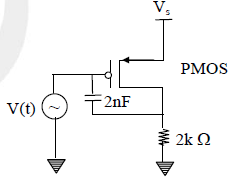(Important - Enter only the numerical value in the answer)

Solution: